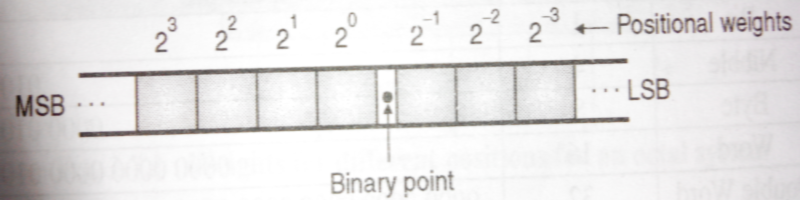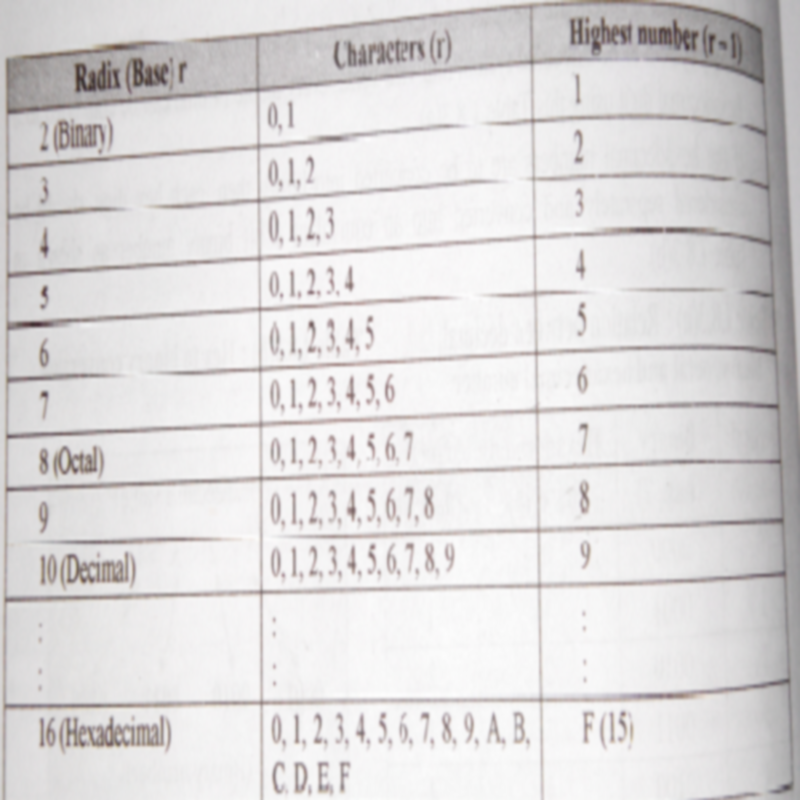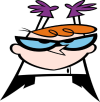## Number System.

To learn various programming languages,we must first learn all types of number system and their conversion.
In this post,i am going to give you some information related to number system.

#### Number system:

A number system defines a set of values used to represent quantity.We talk about the number of people attending class,the number of modules taken per student and also use numbers to represent grades achieved by students in test.Study of number system is not just limited to computers.We apply numbers everyday and knowing how numbers work will give us an insight into how a computer manipulates and stores numbers.
• The number of values that a digit can assume is equal to the base of the system.It is also called as the Radix of system.For example,for a decimal system(0-9),the base is "10",hence every digit can assume 10 values.
• The largest value of a digit is always one less than the base.For example,the largest digit in a decimal system is 9(one less than the base).
• Each digit position represents a different multiple of base,i.e. the numbers have positional importance.
• For example consider the decimal number (349.25)10.Here,the position of each digit is represented as follows:   3*102+4*101+9*100+2*10-1+5*10-2,which is equal to 300+40+9+0.25=349.25.
• Hence,we can implement a common rule for all the numbering system as follows.For a general number,we have to multiply each of digit by some power of base(B) or radix.

#### Various Numbering System:

Name of number system                            Base

1. Binary                                                         2
2. Octal                                                           8
3. Decimal                                                     10
4. Duodecimal                                               12
There are many more number system,but the above listed number system are common and important number system which are used now a days in our day to day life.

### Decimal Number System:

g
The number  system we have been taught to use since school is called as decimal number system.We are familiar with counting and mathematics that uses this system.
Characteristics of a decimal system:
Some of important characteristic of a decimal system are:
1. It uses the base of 10.
2. The largest value of digit is9.
3. Each place represents a different multiple of 10.The multiples are also called as weighted values.The weighted values of each position are as shown below:#### Most Significant Digit(MSD):

It is the leftmost digit having the highest weight.

#### Least Significant Digit(LSD):

It is the rightmost digit having lowest weight.

### The Binary Number System:

• Most modern computer system operate using the binary logic.A computer cannot operate on the decimal number system.
• A binary number system uses only two values 0(for OFF) and 1(for ONN).
• The binary number system works loke a decimal number system except one change.It uses the base 2.
• Hence,the largest value of a digit is 1 an the number of values a digit can assume is two i.e. 0 and 1.
• The weighted values for different positions for a binary system are as show in below fig.• The binary digit(0 and 1) are also called as bits.Thus,binary system is a two bit system.
• The leftmost bit in a given binary number with the highest weight is called as Most Significant Bit(MSB) whereas the rightmost bit in a given number with the lowest weight is called as Least Significant Bit(LSB).

#### Binary Number Format:

• We typically write binary number as a sequence of bits(bits is short for binary digits.).We have definition for there bits.These boundaries are:• In any number base,we may add as many leading zeros as we wish without changing its value.
• However,we normally add leading zeros to adjust the binary number to a desired size of boundary.
• For example,we can represent the number five as:#### Disadvantages of the Binary System:

• The binary number system has an important drawback.It requires a very long string of 1's and 0's to represent a decimal number.For example:
(128)10=(10000000)2.

• So we require 8 bits to represent (128)10.
• To overcome this the other numbering system were developed.

### Octal Number System:

The important features of the octal number system are as follows:
1. Base:The base used for octal number system is 8.
2. Each digit in octal system will assume 8 different values from 0 to 7.
3. The largest value of a digit in the octal system will be 7.The below figure represents the octal number system.The last row of figure represents the largest value of digit.4. Each digit has a different multiple of base.

#### Application  of Octal System:

1. In order to represent large numbers,we will need a huge strings of 0's and 1's if the binary system is used.
2. So the octal system can be used for reducing the size of the large binary number.
3. But it is important to note that the digital circuits and computers internally work strictly with the binary structure and not with the octal structure.
4. The octal structure is used only for the convenience of the user.

The important features of a hexadecimal number system are as follows:
• Base:The base of hexadecimal system is 16.
• The number of values assumed by each digit is 16.The values include digits 0 through 9 followed by alphabets A to F(A,B,C,D,E,F).
• O represent least significant digit whereas F represents the most significant digit.
To have base 16,we need 16 digits.Hence,we use 0 through 9 but need more 6 digits.To overcome this,we use first six alphabets letters A,B,C,D,E,F.Here, A represents 10,B represents 11,C represents 12,D represents 13,E represents 14,F represents 15.

### Counting in a general Radix(Base) r:• We have discussed the number system with radix 10,2,8 and 16.Every such system having a radix "r" contains "r" distinct characters.
• In decimal system the number character is 10.Similarly,in octal system the number of characters is 8.
Check out this video by Soham Railkar.The video is about Introduction to Number System.You can find this video also on SKRtutorials.

### Next update will be related to conversion of number system.

•••